# 超声外科手术系统输出声功率的测量方法研究Measurement of Acoustic Output Poweron Ultrasonic-Surgical Systems

• 全文下载: PDF(738KB)    PP.351-357   DOI: 10.12677/APP.2017.712043
• 下载量: 706  浏览量: 1,312

This paper reviews the principle, method, importance and necessity of testing the output power of ultrasonic surgical system, and uses the hydrophone method to measure the output sound power of the ultrasonic hemostatic knife system. The experimental data are analyzed and discussed. The measurement method and experimental results are of great significance to evaluate the safety and efficacy of ultrasound surgical system.

1. 概述

2. 测量方法

2.1. 测量原理

2.2. 测量实验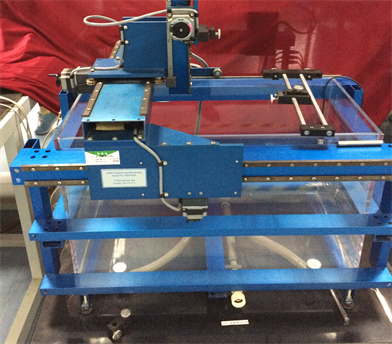Figure 1. AIMS III acoustic intensity measurement system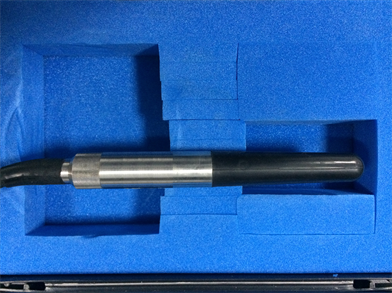Figure 2. RHSA-10 hydrophone

${P}_{ad}=\frac{2\text{π}{r}^{2}|p{\left(r\right)}^{2}|}{\rho c}$ (1)

$p\left(r\right)=\frac{V}{{M}_{L}}$ (2)

${P}_{ad}=\frac{2\text{π}{r}^{2}{V}^{2}}{\rho c{M}_{L}^{2}}$ (3)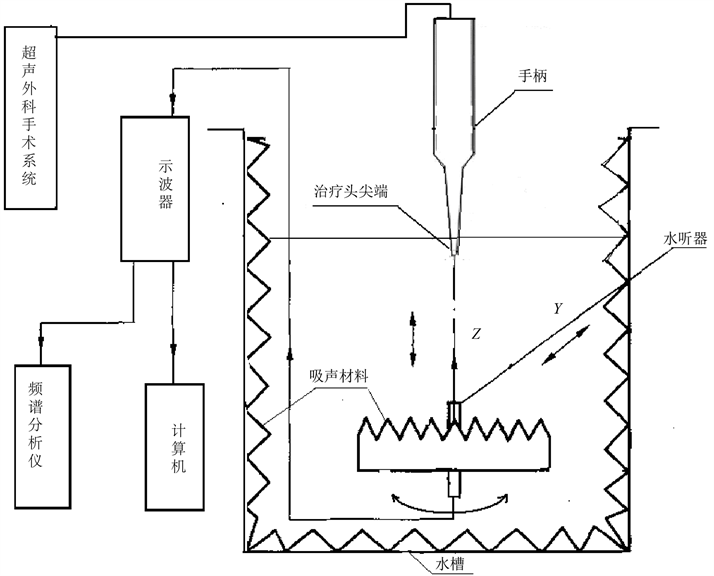Figure 3. Test configuration

${P}_{ad}=\frac{4\text{π}{r}^{2}Vrm{s}^{2}}{\rho c{M}_{L}^{2}}$ (4)

${P}_{ad}=\frac{\text{π}{r}^{2}|p{\left(r\right)}^{2}|}{2\rho c}$ (5)

${P}_{ad}=\frac{\text{π}{r}^{2}{V}^{2}}{2\rho c{M}_{L}^{2}}$ (6)

3. 实验结果与讨论

3.1. 实验数据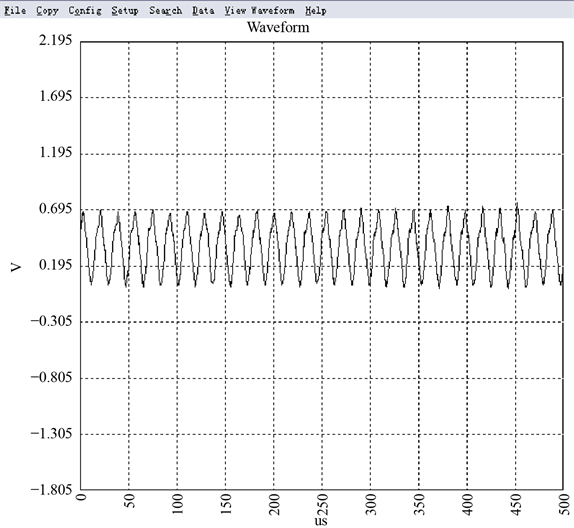Figure 4. Transmitted waveform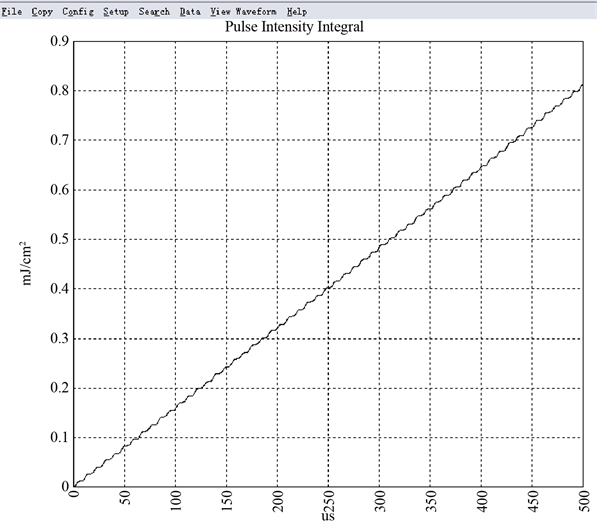Figure 5. Waveform integral

1) 40 k的超声吸引刀具

ρ = 1000 kg/m3, c = 1500 m/s, r = 3.1 cm = 0.031 m,

VRMS = 0.997 V, ML = 2.0892 × 10−5 V/Pa, 根据标准要求，则有：

${P}_{ad}=\frac{4\text{π}{r}^{2}Vrm{s}^{2}}{\rho c{M}_{L}^{2}}=\frac{4×\text{π}×{0.031}^{2}×{\left(0.997\right)}^{2}}{1000×1.5×{10}^{3}×{\left(2.0892×{10}^{-5}\right)}^{2}}=17.16\text{\hspace{0.17em}}\text{W}$

2) 55 k的超声止血刀具

ρ = 1000 kg/m3, c = 1500 m/s, r = 3.0 cm = 0.030 m,

VRMS = 0.997 V, ML = 1.9275 × 10−5 V/Pa, 根据标准要求，则有：

${P}_{ad}=\frac{4\text{π}{r}^{2}Vrm{s}^{2}}{\rho c{M}_{L}^{2}}=\frac{4×\text{π}×{0.030}^{2}×{\left(0.418\right)}^{2}}{1000×1.5×{10}^{3}×{\left(1.9275×{10}^{-5}\right)}^{2}}=3.78\text{\hspace{0.17em}}\text{W}$

3) 40 K超声切骨刀具

ρ = 1000 kg/m3, c = 1500 m/s, r = 3.2 cm = 0.032 m,

VRMS = 0.893 V, ML = 2.0892 × 10−5 V/Pa, 根据标准要求，则有：

${P}_{ad}=\frac{4\text{π}{r}^{2}Vrm{s}^{2}}{\rho c{M}_{L}^{2}}=\frac{4×\text{π}×{0.032}^{2}×{\left(0.893\right)}^{2}}{1000×1.5×{10}^{3}×{\left(2.0892×{10}^{-5}\right)}^{2}}=15.66\text{\hspace{0.17em}}\text{W}$

3.2. 结果汇总

3.3. 结果讨论

  尚志远. 检测声学原理及应用[M] 冯若, 王智彪. 实用超声治疗学[M]. 北京: 科学技术文献出版社, 2002.  Takahashi, H. and Murakami, E. (2010) Ultrasonic Surgical System. Digestive Endoscopy, 14, 163-166.  EPL/87 (1998) Ultrasonics-Surgical Systems-Measurement and Declaration of the Basic Output Characteristics. BS EN 61847:1998, IEC 61847:1998.  . 西安: 西北大学出版社, 1996.  国家武汉医用超声波仪器质量监督检测中心. YY/T 0644-2008超声外科手术系统基本输出特性的测量和公布[S]. 北京: 中国国家标准化管理委员会, 2008.  Petošić, A.I., Jambrošić, B., et al. (2007) Measuring Output Acoustic Power of an Ultrasonic Surgical System Using the Calorimetric Method; Proceedings of the International Congress on Acoustics Official Publication. 19th International Congress on Acoustics, Official Publication.  ONDA (2015) Hydrophone Handbook Datasheet. ONDA, USA, 33.  上海交通大学, 等. GB/T 19890-2005声学 高强度聚焦超声(HIFU)声功率和声场特性的测量[S]. 北京: 中国国家标准化管理委员会, 2005.  Kollmann, C., Haar, G.T., Doležal, L., et al. (2005) Ultrasound, Output, Safety, Thermal Index, Mechanical Index. Journal of the American Society of Echocardiography, 9, 216-220.